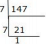# HCF And LCM - MCQ 2

## 20 Questions MCQ Test Quantitative Techniques for CLAT | HCF And LCM - MCQ 2

Description
Attempt HCF And LCM - MCQ 2 | 20 questions in 15 minutes | Mock test for Quant preparation | Free important questions MCQ to study Quantitative Techniques for CLAT for Quant Exam | Download free PDF with solutions
QUESTION: 1

### A rectangular courtyard 140cm long 525cm wide is to be paved exactly with square  tiles, all of the same size. what is the largest size of the tile which could be used for the purpose?

Solution:

30 cm = 4 × 5 × 7
525 cm = 5 × 5 × 3 × 7
Hence common factors are 5 and 7
Hence LCM = 5 × 7 = 35

QUESTION: 2

### Two containers contain 50 and 125 litres of water respectively. Find the maximum capacity of a container which can measure the water in each container an exact number of times(in litres)

Solution:

HCF of 50 and 125 = 25

QUESTION: 3

### Two baskets contain 183 and 242 Apples respectively, which are distributed in equal number among children. Find the largest number of apples that can be given, so that 3 apples are left over from the first basket and 2 from the second.

Solution:

183-3 = 180
142-2 = 240
HCF of 240, 180 = 60

QUESTION: 4

A person has 3 bars whose length are 12,16,24m respectively. He want to cuts the longest possible pieces, all of the same length from each of the 3 bars, what is the length of the each piece, if he is cut without any wastage

Solution:

HCF of 12, 16, 24 = 4m

QUESTION: 5

Four bells commence tolling together and toll at the intervals of 3,9,12,15 seconds resp. In 60 minutes how many times they will toll together.

Solution:

LCM of 3, 9, 12, 15 = 180s = 3m
In 60m = 60/3 = 20⇒ 20+1 = 21

QUESTION: 6

In a seminar the number of participants in Technology, Economics and Science are 150, 90 and 180 respectively. Find the minimum number of rooms required, where in each room the same number of participants are to be seated and all of them being in the same subject.

Solution:

HCF of 150, 90 and 180 = 30
No of participants can be seated in each room = 30

There are total 420 participants.

As the HCF is 30 i.e. mininum number of participants in each room.

so total rooms required will be 420/30 = 14

QUESTION: 7

Naren, Suraj and Praveen start running around a circular stadium and complete one round in 12s, 9s and 15s respectively. In how much time will they meet again at the starting point ?

Solution:

LCM of 12, 9, 15 =180s = 3m

QUESTION: 8

Three boxes of lengths 60m,30m and 45m are to be cut into pieces of equal length. What is the greatest possible length of each pieces ?

Solution:

HCF of 60, 30 and 45m = 15m

QUESTION: 9

In a college, all the students can stand in a row, so that each row has 9, 7 and 12 students. Find the least no of students in the school ?

Solution:

LCM of 9, 7 and 12 = 252

QUESTION: 10

The HCF of 3 different no is 17, Which of the following cannot be their LCM ?

Solution:

To check whether the given number is it's LCM or not just divide the LCM by HCF .

1) 540 ÷ 17 = 31.76

2) 289 ÷ 17 = 17

3) 340 ÷ 17 = 20

4) 425 ÷ 17 = 25 .

540 is not divisible by 17.

Hence : 540 is not the LCM .

QUESTION: 11

If the product of two numbers is 2496 and HCF is 8,then the ratio of HCF and LCM is

Solution:

HCF = 8
LCM = Product/HCF Product of the 2 number = 2496
HCF:LCM = 8 : (2496/8) ⇒ 8 : 312 ⇒ 1: 39

QUESTION: 12

The greatest possible length which can be used to measure exactly the lengths 1m 92cm,3m 84cm ,23m 4cm

Solution:

192 = 42×22×3
384 = 42×22×6
2304 = 42×2×62
HCF = 42×2 = 16×2 = 32

QUESTION: 13

HCF of 4/3, 8/6, 36/63 and 20/42

Solution:

HCF of numerator(4,8,36,20) = 4
LCM of denominator(3,6,63,42) = 126

QUESTION: 14

Find the LCM of 3/8, 9/32, 33/48, 18/72

Solution:

LCM of numerator(3,9,33,18) = 198
HCF of denominator(8,32,48,72) = 8

QUESTION: 15

The HCF of 2511 and 3402 is

Solution:

2511 = 81×31
3402 = 81×42
Hence HCF is 81

QUESTION: 16

A gardener had a number of shrubs to plant in rows. At first he tried to plant 8,then 12 and then 16 in a row but he had always 3 shrubs left with him. On trying 7 he had none left. Find the total number of shrubs.

Solution:QUESTION: 17

If m and n are two whole numbers and if m= 49. Find n^m, given that n ≠ 1

Solution:

49 = 72 = mn
nm = 27 = 128

QUESTION: 18

What will be the least number which when doubled will be exactly divisible by 12,18,21 and 30?

Solution:

LCM of 12,18,21 and 30 = 1260
Doubled (divide by 2) = 1260/2 = 630

QUESTION: 19

HCF and LCM of two numbers are 11 and 385 .If one number lies between 75 and 125 then that number is

Solution:

Product of the two number = 11a×11b = 4235
11a×11ab = 4235/121 = 35
35 = 7 × 5 (co prime)
77 × 11 = 77

QUESTION: 20

If the L.C.M of x and y is z, their H.C.F is

Solution:

HCF = Product of the 2 number/LCM = XY/ZUse Code STAYHOME200 and get INR 200 additional OFF Use Coupon Code